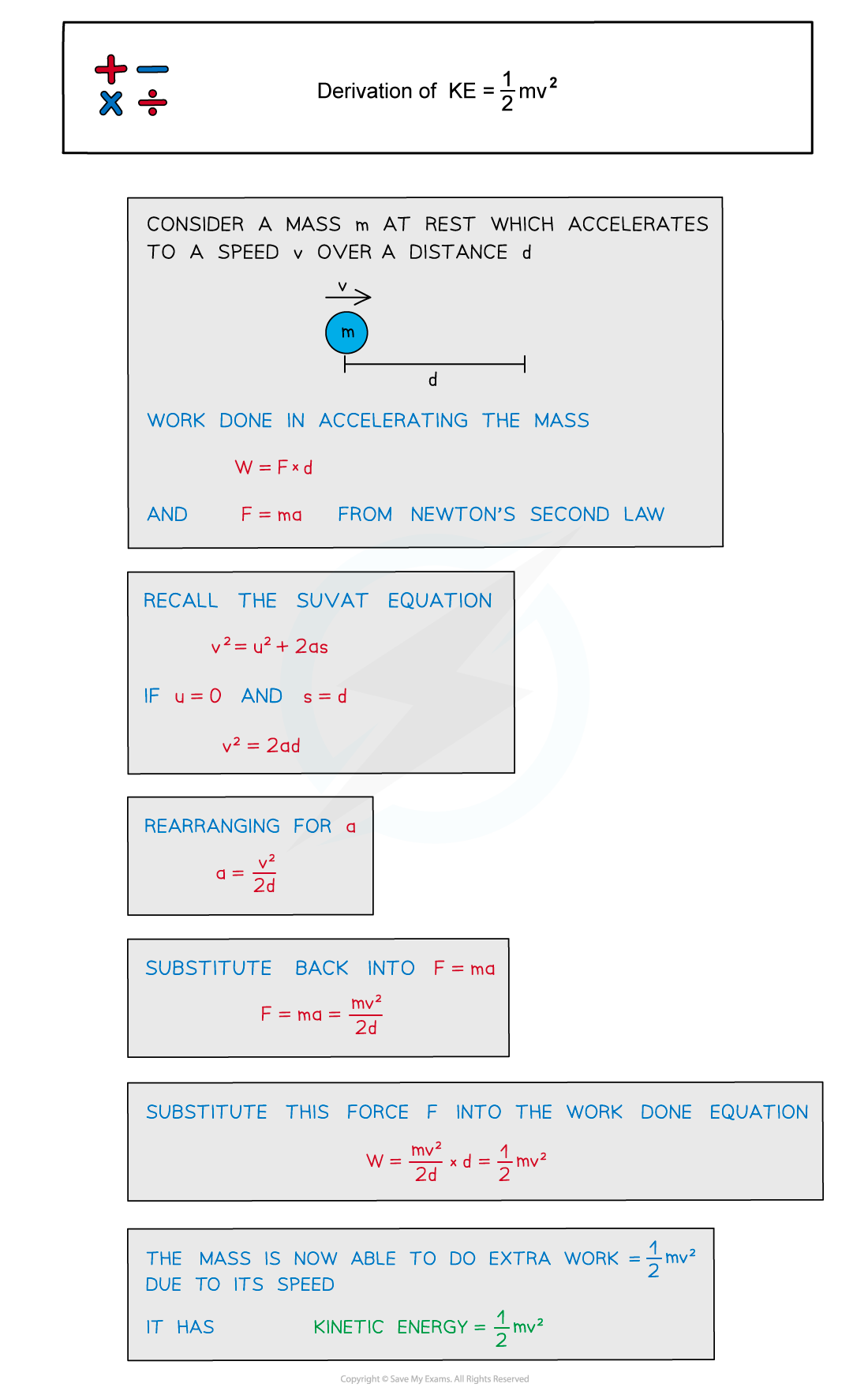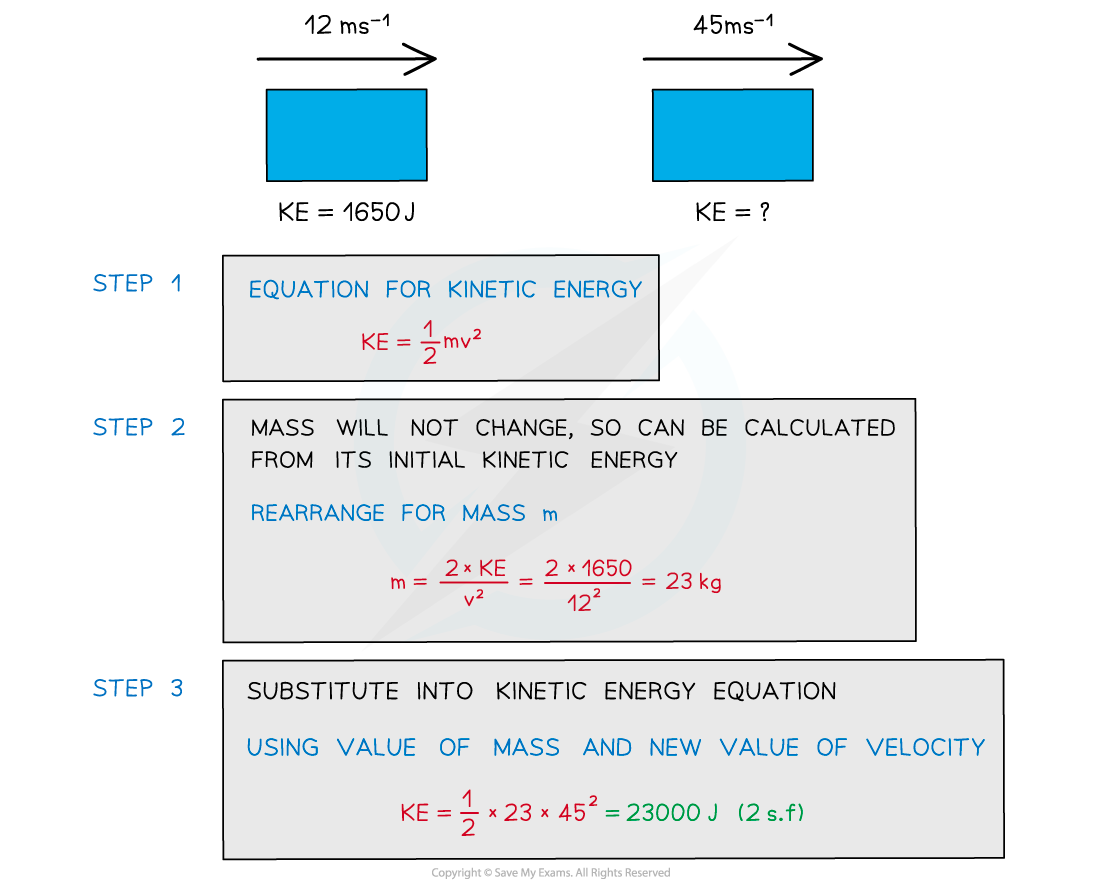# IB DP Physics: SL复习笔记2.3.1 Kinetic Energy

### Kinetic Energy

• Kinetic energy (Ek) is the energy an object has due to its motion (or velocity)
• The faster an object is moving, the greater its kinetic energy
• When an object is falling, it is gaining kinetic energy since it is gaining speed
• This energy transferred from the gravitational potential energy it is losing
• An object will maintain this kinetic energy unless its speed changes
• Kinetic energy can be calculated using the following equation:Kinetic energy (KE): The energy an object has when it is moving

#### Derivation of Kinetic Energy Equation

• A force can make an object accelerate; work is done by the force and energy is transferred to the object
• Using this concept of work done and an equation of motion, the extra work done due to an object's speed can be derived
• The derivation for this equation is shown below:#### Worked Example

A body travelling with a speed of 12 m s–1 has kinetic energy 1650 J.If the speed of the body is increased to 45 m s–1, estimate what is its new kinetic energy.#### Exam Tip

When using the kinetic energy equation, note that only the speed is squared, not the mass or the ½.If a question asks about the ‘loss of kinetic energy’, remember not to include a negative sign since energy is a scalar quantity.